# Diagnostics part 2: error covariance assumptions

10/21/2020

## Instructions:

Use the left and right arrow keys to navigate the presentation forward and backward respectively. You can also use the arrows at the bottom right of the screen to navigate with a mouse.

FAIR USE ACT DISCLAIMER:
This site is for educational purposes only. This website may contain copyrighted material, the use of which has not been specifically authorized by the copyright holders. The material is made available on this website as a way to advance teaching, and copyright-protected materials are used to the extent necessary to make this class function in a distance learning environment. The Fair Use Copyright Disclaimer is under section 107 of the Copyright Act of 1976, allowance is made for “fair use” for purposes such as criticism, comment, news reporting, teaching, scholarship, education and research.

## Outline

• The following topics will be covered in this lecture:
• A review of the residuals and their covariance
• Visual diagnostics of non-constant variances
• The F-test for non-equal variances
• Correlation between cases
• The Durbin-Watson test for time correlation

## Diagnostics

• We are considering 4 categories of potential issues with the model:
1. Issues with the Gaussian error assumption: our hypothesis testing studied thus far relies on the Gaussian error assumption.
2. Issues with the form of the hypothetical covariance: we have assumed that $\mathrm{cov}\left(\mathbf{Y}\right) = \sigma^2\mathbf{I}$ but for many cases this will not be true.
3. Issues with unusual observations: some of the observations may not look like others, and they might change the choice and the fit of the model.
4. Issues with the systematic part of the model: we have assumed that there is an actual signal in the data of the form \begin{align} \mathbb{E}[\mathbf{Y}] = \mathbf{X} \boldsymbol{\beta}, \end{align} which may not be valid.

## Checking error covariance assumptions

• We will now begin our discussion on diagnosing issues with our error covariance assumptions.

• If we wish to check the assumptions on the error or variation in the signal $$\boldsymbol{\epsilon}$$, we need to consider, $$\boldsymbol{\epsilon}$$ itself is not observable.

• Q: What proxy could we consider for the error?

• A: The residuals are related to the error functionally, but have slightly different properties.
• Recall, the definition of $$\hat{\mathbf{Y}}$$

\begin{align} \hat{\mathbf{Y}} \triangleq& \mathbf{X}\left(\mathbf{X}^\mathrm{T} \mathbf{X}\right)^{-1} \mathbf{X}^\mathrm{T} \mathbf{Y} \\ & =\mathbf{H} \mathbf{Y} \end{align}

• Therefore, if we compute the residuals,

\begin{align} \hat{\boldsymbol{\epsilon}} & = \mathbf{Y} - \hat{\mathbf{Y}} \\ & =\left(\mathbf{I} - \mathbf{H}\right)\mathbf{Y} \\ & =\left(\mathbf{I} - \mathbf{H}\right)\mathbf{X} \boldsymbol{\beta} + \left(\mathbf{I} - \mathbf{H}\right)\boldsymbol{\epsilon} \end{align}

### Checking error covariance assumptions

• From the last slide

\begin{align} \hat{\boldsymbol{\epsilon}} & =\left(\mathbf{I} - \mathbf{H}\right)\mathbf{X} \boldsymbol{\beta} + \left(\mathbf{I} - \mathbf{H}\right)\boldsymbol{\epsilon} \end{align}

• Q: recalling the definition of $$\mathbf{H} = \mathbf{X}\left(\mathbf{X}^\mathrm{T} \mathbf{X}\right)^{-1} \mathbf{X}^\mathrm{T}$$, what does $$\mathbf{H}\mathbf{X}$$ equal to?

• A: $$\mathbf{H}$$ is the projection operator onto the span of the design matrix, and thus $$\mathbf{H}\mathbf{X} = \mathbf{X}$$ by construction.

• Q: given the above, what does $$\left(\mathbf{I} - \mathbf{H}\right)\mathbf{X}$$ equal to?

• A: the above must equal zero, as $$\mathbf{I}\mathbf{X} = \mathbf{H}\mathbf{X} = \mathbf{X}$$.

### Checking error covariance assumptions

• From the previous two exercises, we can deduce,

\begin{align} \hat{\boldsymbol{\epsilon}} = \left(\mathbf{I} - \mathbf{H}\right)\boldsymbol{\epsilon} \end{align}

• We take the assumption that $$\boldsymbol{\epsilon}\sim N(0, \mathbf{I} \sigma^2)$$,

• Q: given the above assumption, what is the mean of $$\hat{\boldsymbol{\epsilon}}$$?
• A:

\begin{align} \mathbb{E}[\hat{\boldsymbol{\epsilon}}] &= \mathbb{E}\left[\left(\mathbf{I} - \mathbf{H}\right)\boldsymbol{\epsilon}\right] \\ &=\left(\mathbf{I} - \mathbf{H}\right)\mathbb{E}\left[\boldsymbol{\epsilon}\right]\\ &= 0 \end{align}

• Q: given the above assumption, what is the covariance of $$\hat{\boldsymbol{\epsilon}}$$?
• A:

\begin{align} \mathbb{E}\left[\left(\hat{\boldsymbol{\epsilon}}\right) \left(\hat{\boldsymbol{\epsilon}}\right)^\mathrm{T}\right] &= \mathbb{E}\left[\left(\left(\mathbf{I} - \mathbf{H}\right)\boldsymbol{\epsilon}\right)\left(\left(\mathbf{I} - \mathbf{H}\right)\boldsymbol{\epsilon}\right)^\mathrm{T}\right] \\ &=\mathbb{E}\left[\left(\mathbf{I} - \mathbf{H}\right)\boldsymbol{\epsilon}\boldsymbol{\epsilon}^\mathrm{T}\left(\mathbf{I} - \mathbf{H}\right)^\mathrm{T}\right]\\ &=\left(\mathbf{I} - \mathbf{H}\right)\mathbb{E}\left[\boldsymbol{\epsilon}\boldsymbol{\epsilon}^\mathrm{T}\right]\left(\mathbf{I} - \mathbf{H}\right)^\mathrm{T}\\ & =\left(\mathbf{I} - \mathbf{H}\right)\left[\sigma^2 \mathbf{I}\right]\left(\mathbf{I} - \mathbf{H}\right)\\ &=\sigma^2\left(\mathbf{I} - \mathbf{H}\right) \end{align}

### Checking error covariance assumptions

• Q: Why is the last slide relevant? Particularly, why should we be concerned with the covariance of the residuals?

• A: If the assumptions hold for $$\boldsymbol{\epsilon}$$, then we can compare the coviance of the residuals to their theoretical value $$\sigma^2\left(\mathbf{I} - \mathbf{H}\right)$$ for consistency.
• Occasionally, we might actually have prior knowledge about the value of $$\sigma^2$$ which we can evaluate directly.
• Otherwise, we have an unbiased estimator for $$\sigma^2$$ in terms of $\hat{\sigma}^2 = \frac{RSS}{n-p}$ which we can compare with the observed covariance of the residuals.
• Note, even though the errors are assumed to be independent and of equal variance $$\sigma^2$$, the same doesn't hold for the residuals.

• In particular, the operator $$\mathbf{I} - \mathbf{H}$$ is not generally diagonal (such that the residuals have correlation); nor are the diagonal values equal (so that the variances don't all match).
• Nonetheless, we use the residuals to underrstand how the true errors are behaving, which are unobservable.

## Visual diagnostics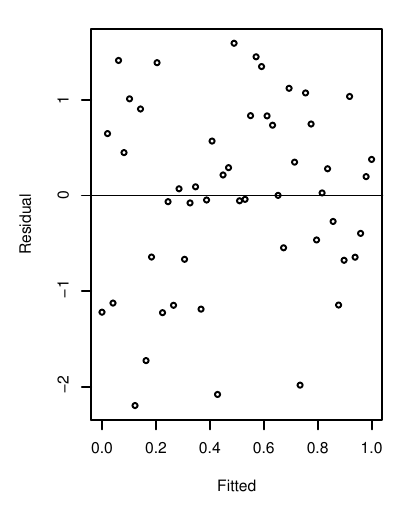Courtesy of: Faraway, J. Linear Models with R. 2nd Edition

• To the left is a plot where:
1. The horizontal (x-axis) represents the fitted value $$\hat{\mathbf{Y}}$$, as in some model $$\hat{\mathbf{Y}}=\mathbf{X}\hat{\boldsymbol{\beta}}$$.
2. The vertical axis (y-axis) represents the corresponding residual $$\hat{\boldsymbol{\epsilon}}$$ value, equal to $$\mathbf{Y} - \hat{\mathbf{Y}}$$.
• Suppose that the variances of the error $$\boldsymbol{\epsilon}$$ are not fixed, i.e.,
• suppose that some observations have more variation and some observations have less variation around the signal, $$\mathbf{X}\boldsymbol{\beta}$$.
• In this case, there is dependence of the variation (or error $$\boldsymbol{\epsilon}$$) on the observation.
• To the left, this is actuall a well behaved situation. In particular, the residuals show variation that doesn’t seem to depend on the value of the fitted value/ observation.
• The mean of the residuals appears to be zero as well, because there isn’t a clear preference for positive or negative residuals
• The situation to the left is denoted homoscedasticity.
• Later, we will develop tests to determine if the variance is “close-enough-to-constant”, so that we are satisfied.
• On the other hand, if there are non-constant variances (as discussed above), this will often show up as a pattern in the plot to the left.

### Visual diagnostics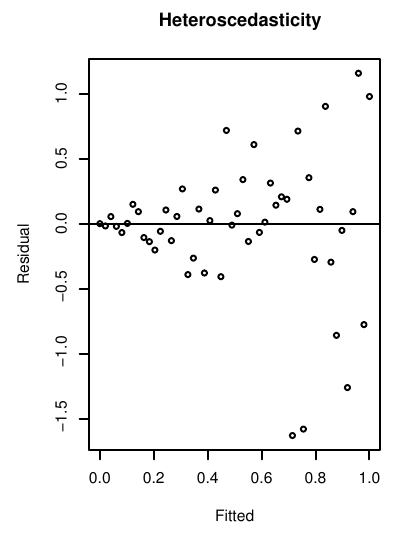Courtesy of: Faraway, J. Linear Models with R. 2nd Edition

• The non-constant variance of $$\hat{\boldsymbol{\epsilon}}$$ to the left is known as heteroscedasticity.
• In this case, there is a clear dependence on the variation of $$\hat{\boldsymbol{\epsilon}}$$ on the fitted value/ the observation.
• Breaking the constant variance assumption, the Gauss-Markov theorem no longer applies
• Heteroscedasticity does not, in itself, cause ordinary least squares coefficient estimates to be biased;
• however, the theoretical estimates of the variance of the residuals (and therefore the standard errors) will become biased.

### Visual diagnosticsCourtesy of: Faraway, J. Linear Models with R. 2nd Edition

• The bias in the standard errors complicates our ability to accurate quantify the uncertainty, and therefore to accurately:
1. make hypothesis tests on the parameters for significance;
2. provide confidence intervals for the parameters
3. provide accurate prediction intervals and confidence intervals for the mean response;
4. provide explanatory power in the relationship between the response and the explanatory variables.

### Visual diagnostics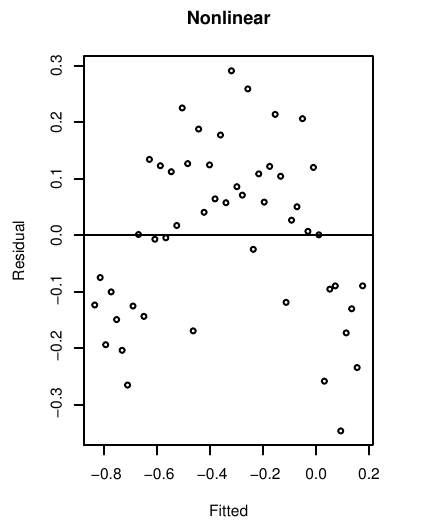Courtesy of: Faraway, J. Linear Models with R. 2nd Edition

• Using the same plot as before, we can likewise determine if there is actually nonlinearity in the residuals.
• The plot at the left exhibits a nonlinear dependence of the residual on the fitted/ observed values
• The same technique can also be used replacing the fitted values $$\hat{\mathbf{Y}}$$ in the horizontal axis with any other variable in the model $$\mathbf{X}_i$$, to determine dependence of the residual on the explanatory variables
• Additionally, we may consider how the residual varies across variables $$\mathbf{X}_i$$ that we have data for, but have not included as explanatory variables on the response.
• If there is a dependence structure for the residuals on the variable that was left out of the model, it suggests that we should consider its impact on the response and/or if it is a variable that is tightly correlated with our existing model variables.

## An example of non-constant variance

• Recall the “savings” dataset, listing the average savings rate, age demographics and per capita incomes of fifty countries averaged over 1960 -1970.
library("faraway")
lmod_savings <- lm(sr ~ pop15+pop75+dpi+ddpi,savings)
sumary(lmod_savings)

               Estimate  Std. Error t value  Pr(>|t|)
(Intercept) 28.56608654  7.35451611  3.8842 0.0003338
pop15       -0.46119315  0.14464222 -3.1885 0.0026030
pop75       -1.69149768  1.08359893 -1.5610 0.1255298
dpi         -0.00033690  0.00093111 -0.3618 0.7191732
ddpi         0.40969493  0.19619713  2.0882 0.0424711

n = 50, p = 5, Residual SE = 3.80267, R-Squared = 0.34

• We saw in the last lecture that the residuals do not diverge strongly from the Gaussian assumption, but some of the predictors themselves appear to have skew or multimodal distributions.

• We will be interested now in examining our error covariance assumptions.

### Savings data example

• With this model, we plot the residuals versus the fitted values:
par(mai=c(1.5,1.5,.5,.5), mgp=c(3,0,0))
plot(fitted(lmod_savings),residuals(lmod_savings),xlab="Fitted",ylab="Residuals", cex=3, cex.lab=3, cex.axis=3)
abline(h=0)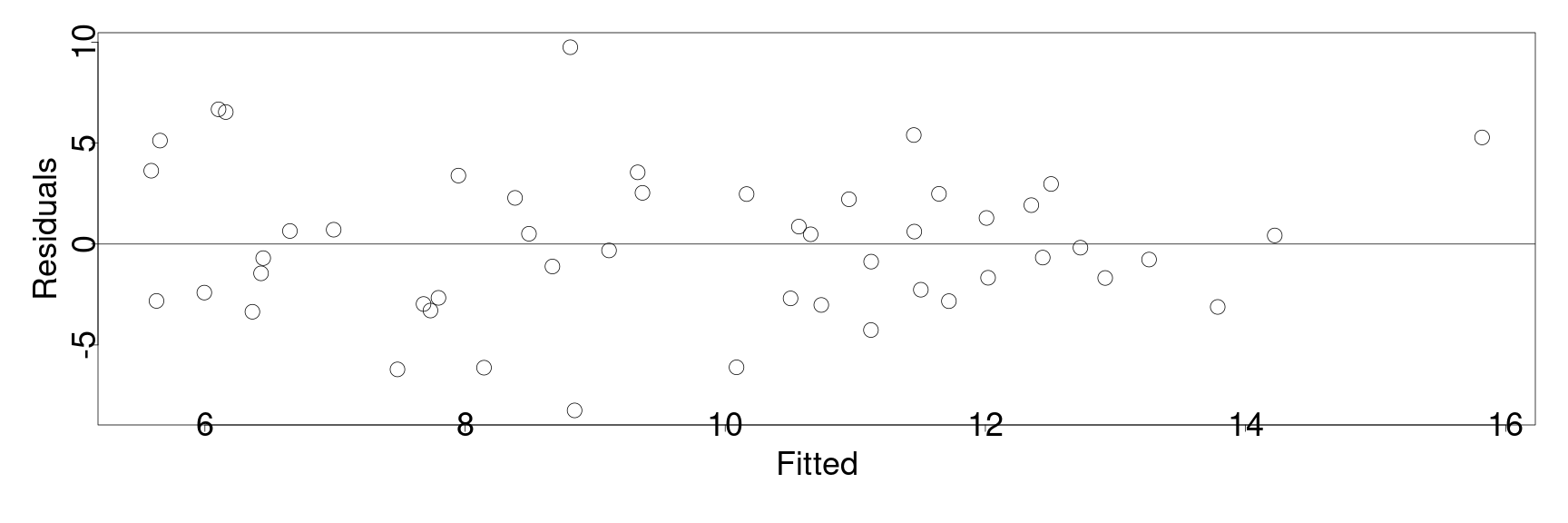• From the initial inspection, we do not notice anything particularly structured about the residuals – indeed they are roughly symmetric around zero and in the fitted value.

### Half normal distributions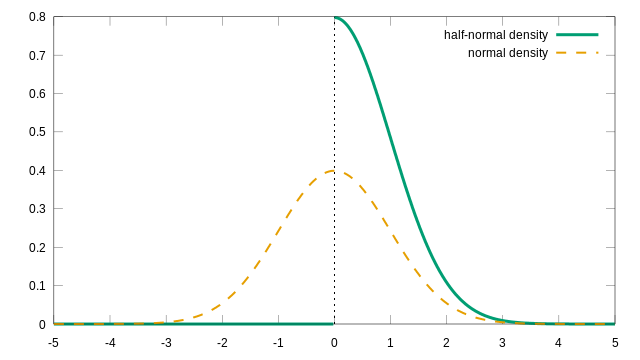Courtesy of Nagelum CC BY-SA 4.0 via Wikimedia Commons
• Therefore, to investigate the constant variance assumption more closely, we will “zoom” in on the residuals.
• In particular, we will consider the plot of the square root of the absolute residuals, i.e., \begin{align} \sqrt{\vert\hat{\boldsymbol{\epsilon}}\vert} \end{align}
• Under the assumption $$\boldsymbol{\epsilon}\sim N(0, \mathbf{I}\sigma^2)$$, the values of $$\vert\hat{\boldsymbol{\epsilon}}\vert$$ are distribted according to the half normal distribution
• the relationship between the normal distribution and the corresponding half-normal distribution is illustrated on the left.
• Having excluded the nonlinearity expressed in other plots, we will focus on the absolute values to increase the resolution of the residuals as a response of the fitted values.
• However, the half normal distribution is very skewed, so we make a change of scale with the square root of the absolute values in order to keep it well behaved.
• ### Savings data example

• In the case below, we plot $$\sqrt{\vert\hat{\boldsymbol{\epsilon}}\vert}$$ versus the fitted values
par(mai=c(1.5,1.5,.5,.5), mgp=c(3,0,0))
plot(fitted(lmod_savings),sqrt(abs(residuals(lmod_savings))), xlab="Fitted",ylab=expression(sqrt(hat(epsilon))), cex=3, cex.lab=3, cex.axis=1.5)